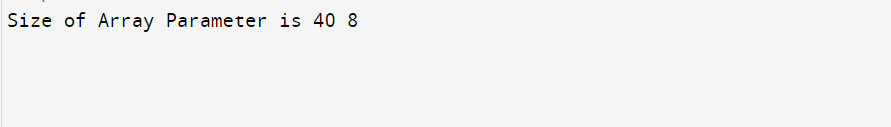top of page
Search

# How to print size of array parameter in C++?

Example:

```#include <iostream>
using namespace std;

void findSize(int arr[])
{
cout << sizeof(arr) << endl;
}

int main()
{
int a;
cout << sizeof(a) << " ";
findSize(a);
return 0;
}```

Output:The above output is for a machine where size of integer is 4 bytes and size of a pointer is 8 bytes.

The cout statement inside main prints 40, and cout in findSize prints 8. The reason is, arrays are always passed pointers in functions, i.e., findSize(int arr[]) and findSize(int *arr) mean exactly same thing. Therefore the cout statement inside findSize() prints size of a pointer.

The Tech Platform